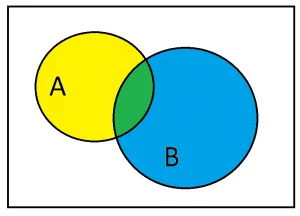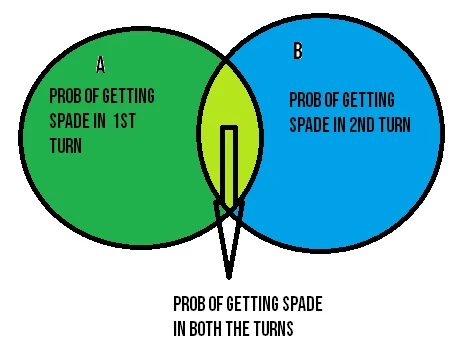25 Feb 2023

Posted on:

04 Jan 2021

0

# Resolved:Homework: Multplication Rule (getting a spade either in the first turn or second turn)

Hi 365 data science team,
I need help with the short homework that is given in the multiplication rule module. So the homework is to find the probability of getting a spade either in the first turn or second turn. I am not entirely sure how to find this probability.
According to my research on google, a person has solved this by calculating the following:
The probability of not getting a spade in the first turn: 39/52
The probability of not getting a spade in the second turn: 38/51
After finding these two probabilities, they simply do the following:
1 - 39*38/52*51 = 0.44
Therefore, there is a 44% probability of drawing a spade either in the first turn or the second turn.
But still, even with this answer, I am not sure If I am following the logic behind this calculation (I don't know whether this follow the additive law or the multiplication rule). Therefore, it would be great if you can explain this in more detail. Please do share if there is an alternative to solve this question.
Jimmy

Posted on:

21 Jan 2021

4

Hi,

I've also struggled getting around this problem, yet I think I've come to understand it a bit better now. The key word here is "either", because it means that the probability of each independent outcome in which a spade has been drawn adds up to the whole probability of getting a spade in whichever of the specified amount of draws (union of events) minus their intersection (to account for double counting). These favorable outcomes end up being:

1. Drawing a spade on the first draw and not drawing one on the second
2. Drawing a spade on both draws
3. Drawing a spade on the second draw and not drawing one on the first

It is quite important to define what our events are, because defining:

• A = drawing a spade on the first draw
• B = drawing a spade on the second draw
• A' = NOT drawing a spade on the first draw
• B' = NOT drawing a spade on the second drawMeans that A = drawing a spade on the first draw and drawing a spade on the first and second draws (because in both outcomes we've drawn a spade on the first draw).

The same can be said about B. B = drawing a spade on the second draw and drawing a spade on the first and second draws (again because in both outcomes we've drawn a spade on the second draw).

Therefore:

• A = A|B' + AnB (yellow + green parts of A in the image)
• B = B|A' + AnB (blue + green parts of B in the image)
• A|B' = previously stated favorable outcome #1 (yellow part in the image)
• AnB = previously stated favorable outcome #2 (green part in the image)
• B|A' = previously stated favorable outcome #3 (blue part in the image)

As I said earlier, these probabilities end up adding up because we're looking for AuB, and:

• AuB = A + B - AnB (Additive law)
• AuB = (A|B' + AnB) + (B|A' + AnB) - AnB
• Aub = A|B' + AnB + B|A' (yellow + green + blue parts in the image = AuB)

Calculating these probabilities:

• P(A) = P(A|B') + P(AnB)
• P(B) = P(B|A') + P(AnB)
• P(A|B') = 13/52 * 39/51 = 507/2652 = 0.191
• P(B|A') = 39/52 * 13/51 = 507/2652 = 0.191
• P(AnB) = 13/52 * 12/51 = 156/2652 = 0.059
• P(AuB) = P(A|B') + P(AnB) + P(B|A')
• P(AuB) = 507/2652 + 156/2652 + 507/2652 = 1170/2652 = 195/442 = 0.191 + 0.059 + 0.191 = 0.441
• P(AuB) = 0.441

As a last note, notice how defining:

• A = drawing a spade only on the first draw (yellow part on the image)
• B = drawing a spade only on the second draw (blue part on the image)

could have totally changed the approach (hence its importance), but the solution would have been the same:

• A + B + AnB = A|B' + B|A' + AnB = 0.441

Regarding the answer you found, it is simply doing the opposite of what I've done. Instead of listing the favorable outcomes as those where we draw a spade, they listed those where we don't. Then they deduct those probabilities from the total probability (100% or 1), ending up with the probability of the unfavorable outcomes (those where we draw a spade). It is not strictly following any of the additive or multiplication rules.

Hope this helps!

Instructor
Posted on:

22 Jan 2021

0
Hey everyone!   Jacobo is bang on with his interpretation and explanation here.    Best,  365 Vik
Posted on:

08 Nov 2022

0

let us use both the multiplicative rule and additive rule to find the answer for this question.
In this question we need to find the probability of getting spades in either first turn or second turn.
let us assume getting spade in first turn as event A and the latter is event B.
A intersection B says getting spades in both the turns.
A|B says the probability of getting spade in 1st turn given getting spade in second turn also

To find the prob of getting spade in either 1st turn or second turn in A union B
so to find this we need to use the additive rule.

``````P(AuB)=P(A)+P(B)-P(AnB)
``````from the diagram,
P(A)=getting spades in 1st turn = 13/52=1/4=0.25
P(B)=getting spades in 2nd turn = 13/52=1/4=0.25
P(A|B)= 12/51
we know that P(AnB)=P(A|B)*P(B)==> 12/51*0.25= 0.05882325294

putting all together in the formula P(AuB)=P(A)+P(B)-P(AnB)
P(AuB)=0.25+0.25-0.05882325294= 0.44117
therefore the probability of getting spades in either of the two turns is 44%.

Posted on:

25 Feb 2023

0

If we put B= getting a spade in the first time

and A= getting a spade in the second time

in order to find the Union of both we use the Additive law, that states:

P(AuB)= P(A)+P(B)-P(AnB)

p(B)= 13/52 = 0.25

p(A)= 13/51 = 0.254

Now in order to find probabilty of their intersection we will use The Multiplication Rule:

P(AnB)= P(A|B)*P(B)

p(A|B)= 12/51= 0.235

p(AnB)= 0.235*0.25= 0.058

now if we plug everything in the Additive law we get:

p(AuB)= 0.254+0.25-0.058= 0.446

and thats the probability to get a spade in wither the first or second time :)